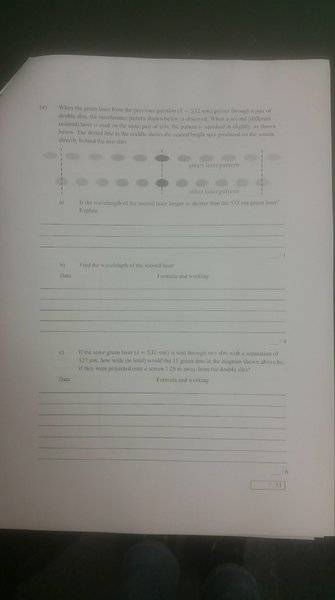# Young's double slit experiment green laser

## Homework Statement

If a green laser is (wavelength = 532nm) sent through two slits with a separation of 127 um, how wide (in total) would the 11 green dots formed be if they were projected onto a screen 1.25m away from the slits?

Refer to this diagram sorry for bad quality: https://imgur.com/a/ACcJYJdmλ=dsinθ
tanθ = Y/L

## The Attempt at a Solution

First find θ

sinθ = mλ/d

sinθ = [(5)(5.32x10^-9)] / 1.27x10^-6
sinθ = 2.09x10^-2
θ = 1.2 degrees

second, find distance from m = 0 to m = 5 (Y)
tanθ = Y/L
Y = Ltanθ
Y = 1.25tan(1.2)
Y = 2.61x10^-2m

So therefore, the total distance of the 11 dots will be twice the distance between M=0 and M=5
Total width of 11 dots = (2.61x10^-2) x 2 = 5.24x10^-2m

ehild
Homework Helper

## Homework Statement

If a green laser is (wavelength = 532nm) sent through two slits with a separation of 127 um, how wide (in total) would the 11 green dots formed be if they were projected onto a screen 1.25m away from the slits?

Refer to this diagram sorry for bad quality: https://imgur.com/a/ACcJYJd

mλ=dsinθ
tanθ = Y/L

## The Attempt at a Solution

First find θ

sinθ = mλ/d

sinθ = [(5)(5.32x10^-9
)] / 1.27x10^-6
sinθ = 2.09x10^-2
θ = 1.2 degrees

second, find distance from m = 0 to m = 5 (Y)
tanθ = Y/L
Y = Ltanθ
Y = 1.25tan(1.2)
Y = 2.61x10^-2m

So therefore, the total distance of the 11 dots will be twice the distance between M=0 and M=5
Total width of 11 dots = (2.61x10^-2) x 2 = 5.24x10^-2m
532 nm is 532x10-9m.
And what is the slit separation? 127 μm or 1.27 μm?

532 nm is 532x10-9m.
And what is the slit separation? 127 μm or 1.27 μm?

127um